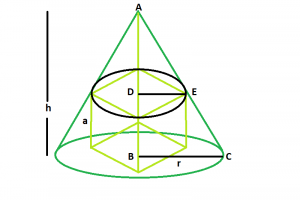GeeksforGeeks App
Open AppBrowser
Continue

# Largest cube that can be inscribed within a right circular cone

Given a right circular cone of radius r and perpendicular height h. We have to find the side length of the biggest cube that can be inscribed within it.
Examples

```Input : h = 5, r = 6
Output : 3.14613

Input : h = 8, r = 12
Output : 5.43698```Approach
Let, side of the cube = a.
From the diagram, we can clearly understand using the properties of triangles: BC/AB = DE/AD.
Therefore,

```r/h = (a/√2)/(h-a)

or, a = h*r√2/(h+√2*r)```

Below is the implementation of the above approach:

## C++

 `// C++ Program to find the biggest cube``// inscribed within a right circular cone``#include ``using` `namespace` `std;` `// Function to find the side of the cube``float` `cubeSide(``float` `h, ``float` `r)``{``    ``// height and radius cannot be negative``    ``if` `(h < 0 && r < 0)``        ``return` `-1;` `    ``// side of the cube``    ``float` `a = (h * r * ``sqrt``(2)) / (h + ``sqrt``(2) * r);` `    ``return` `a;``}` `// Driver code``int` `main()``{``    ``float` `h = 5, r = 6;` `    ``cout << cubeSide(h, r) << endl;` `    ``return` `0;``}`

## Java

 `// Java Program to find the biggest cube``// which can be inscribed within a right circular cone` `import` `java.io.*;` `class` `GFG {`  `// Function to find the side of the cube` `static` `float` `cube(``float` `h, ``float` `r)``{` `    ``// height and radius cannot be negative``    ``if` `(h < ``0` `&& r < ``0``)``        ``return` `-``1``;` ` ``// side of the cube``    ``float` `a = (h * r * (``float``)Math.sqrt(``2``)) / (h + (``float``)Math.sqrt(``2``) * r);``  ` `    ``return` `a;``}` `// Driver code``  ` `    ``public` `static` `void` `main (String[] args) {``          ``float` `h = ``5``, r = ``6``;``    ``System.out.println( cube(h, r));``    ``}``}` `// this article is contributed by Ishwar Gupta`

## Python 3

 `# Python3 Program to find the biggest cube``# inscribed within a right circular cone``import` `math` `# Function to find the side of the cube``def` `cubeSide(h, r):` `    ``# height and radius cannot``    ``# be negative``    ``if` `(h < ``0` `and` `r < ``0``):``        ``return` `-``1` `    ``# side of the cube``    ``a ``=` `((h ``*` `r ``*` `math.sqrt(``2``)) ``/``         ``(h ``+` `math.sqrt(``2``) ``*` `r))` `    ``return` `a` `# Driver code``h ``=` `5``; r ``=` `6``;` `print``(cubeSide(h, r), ``"\n"``)` `# This code is contributed``# by Akanksha Rai`

## C#

 `// C# Program to find the ``// biggest cube which can be``// inscribed within a right``// circular cone``using` `System;` `class` `GFG``{` `// Function to find the side``// of the cube``static` `float` `cube(``float` `h, ``float` `r)``{` `// height and radius cannot be negative``if` `(h < 0 && r < 0)``    ``return` `-1;` `// side of the cube``float` `a = (h * r * (``float``)Math.Sqrt(2)) /``          ``(h + (``float``)Math.Sqrt(2) * r);` `    ``return` `a;``}` `// Driver code``public` `static` `void` `Main ()``{``    ``float` `h = 5, r = 6;``    ``Console.Write( cube(h, r));``}``}` `// This code is contributed``// by 29AjayKumar`

## PHP

 ``

## Javascript

 ``

Output:

`3.14613`

Time Complexity: O(1)

Auxiliary Space: O(1), since no extra space has been taken.

My Personal Notes arrow_drop_up